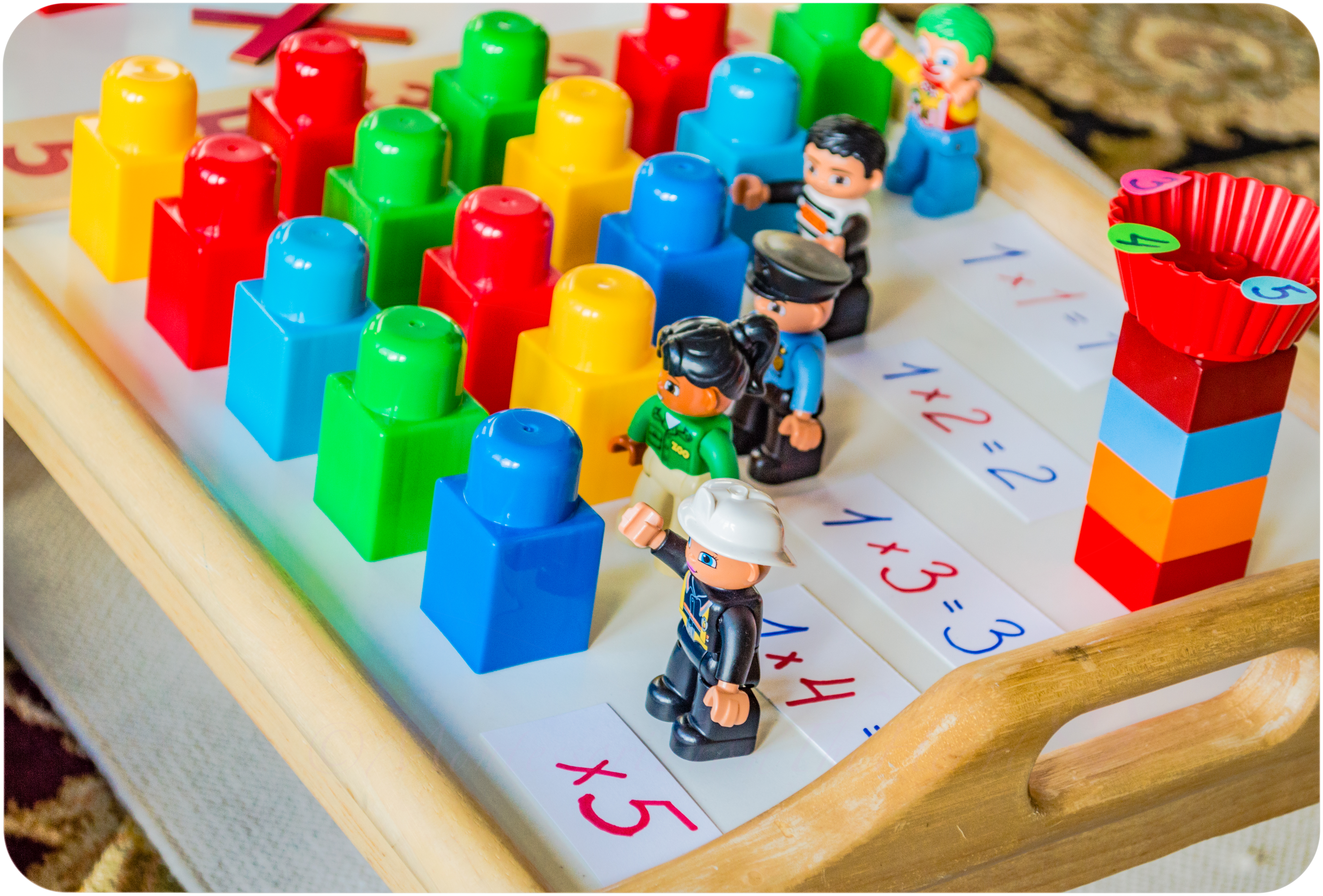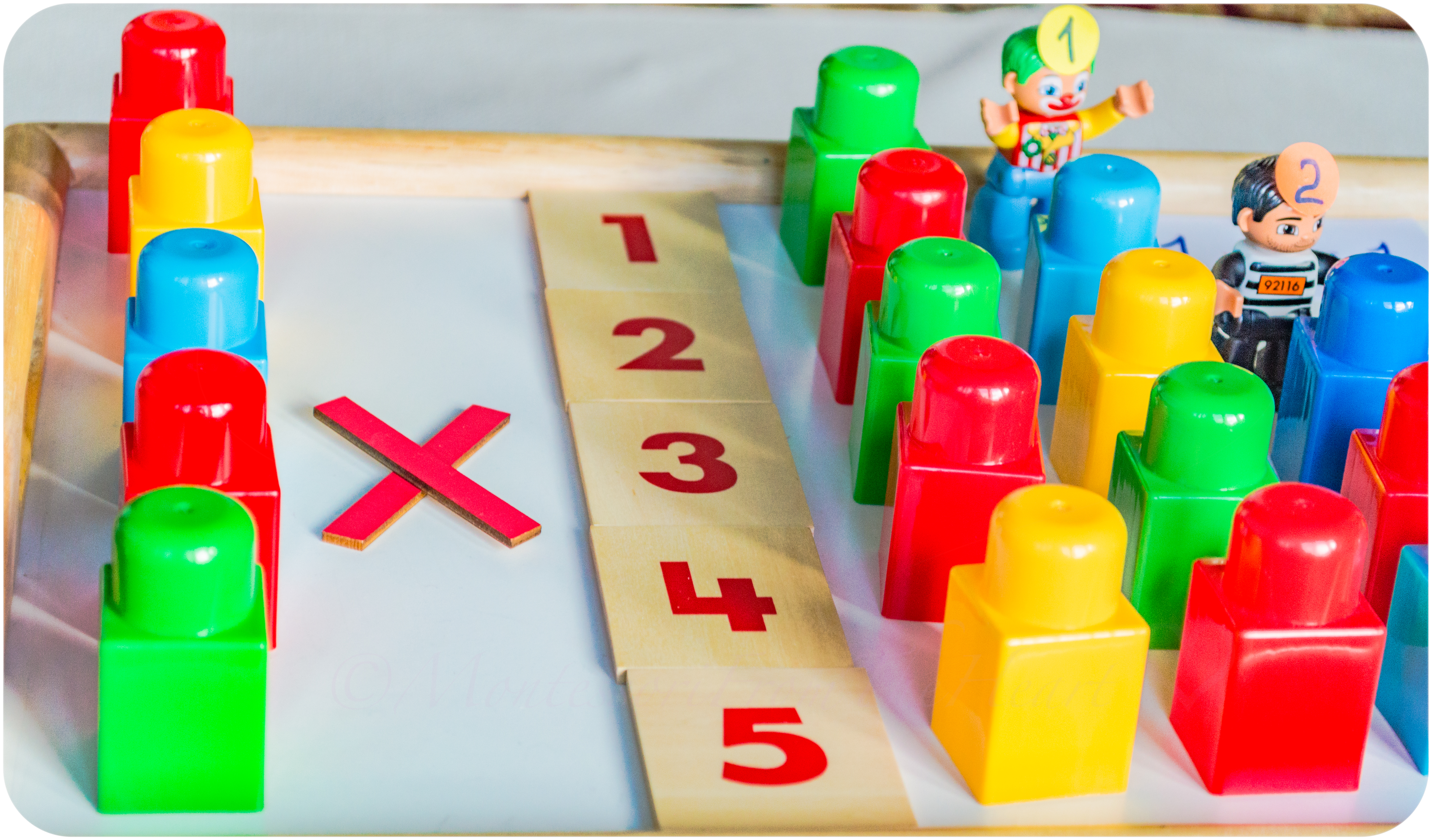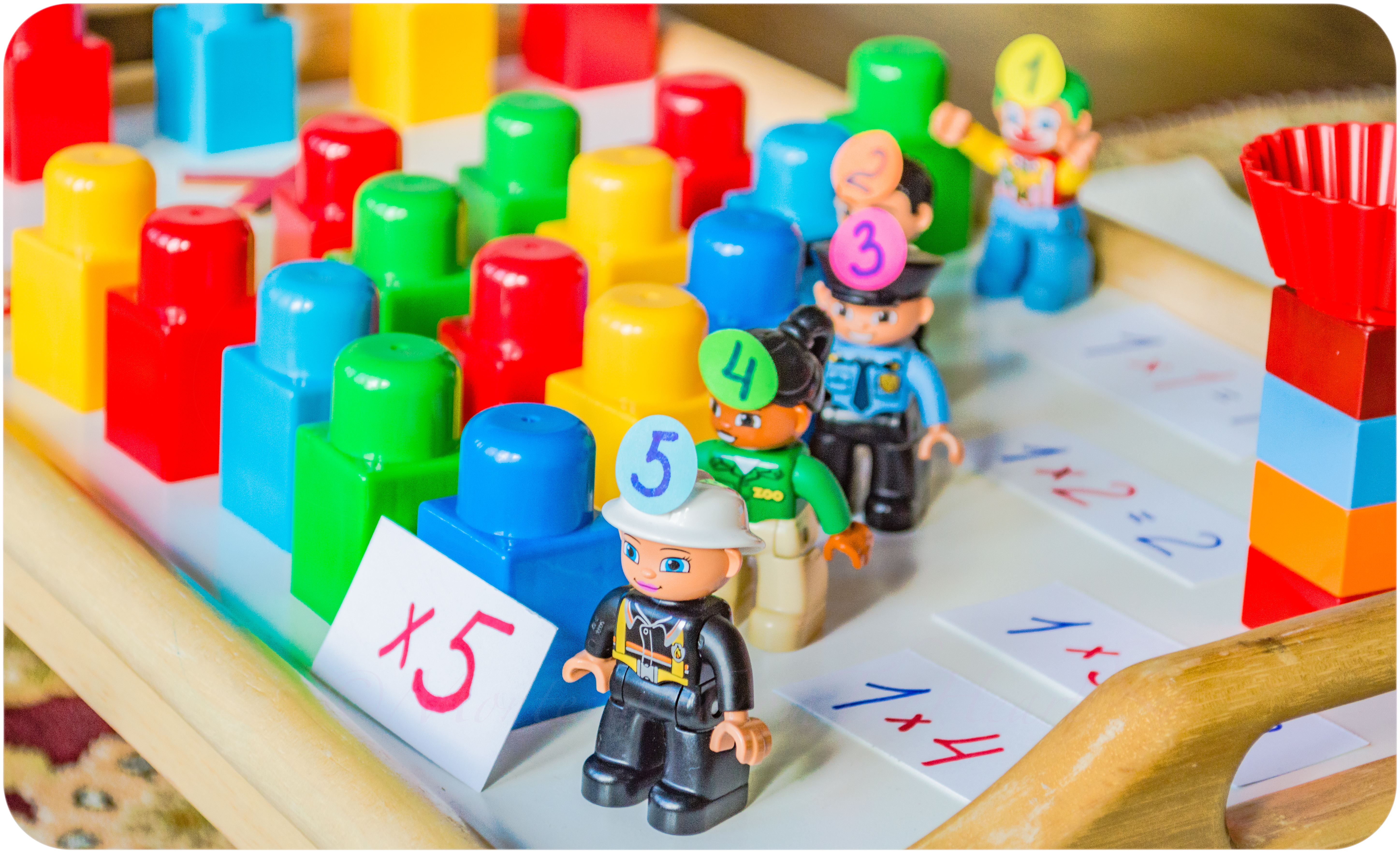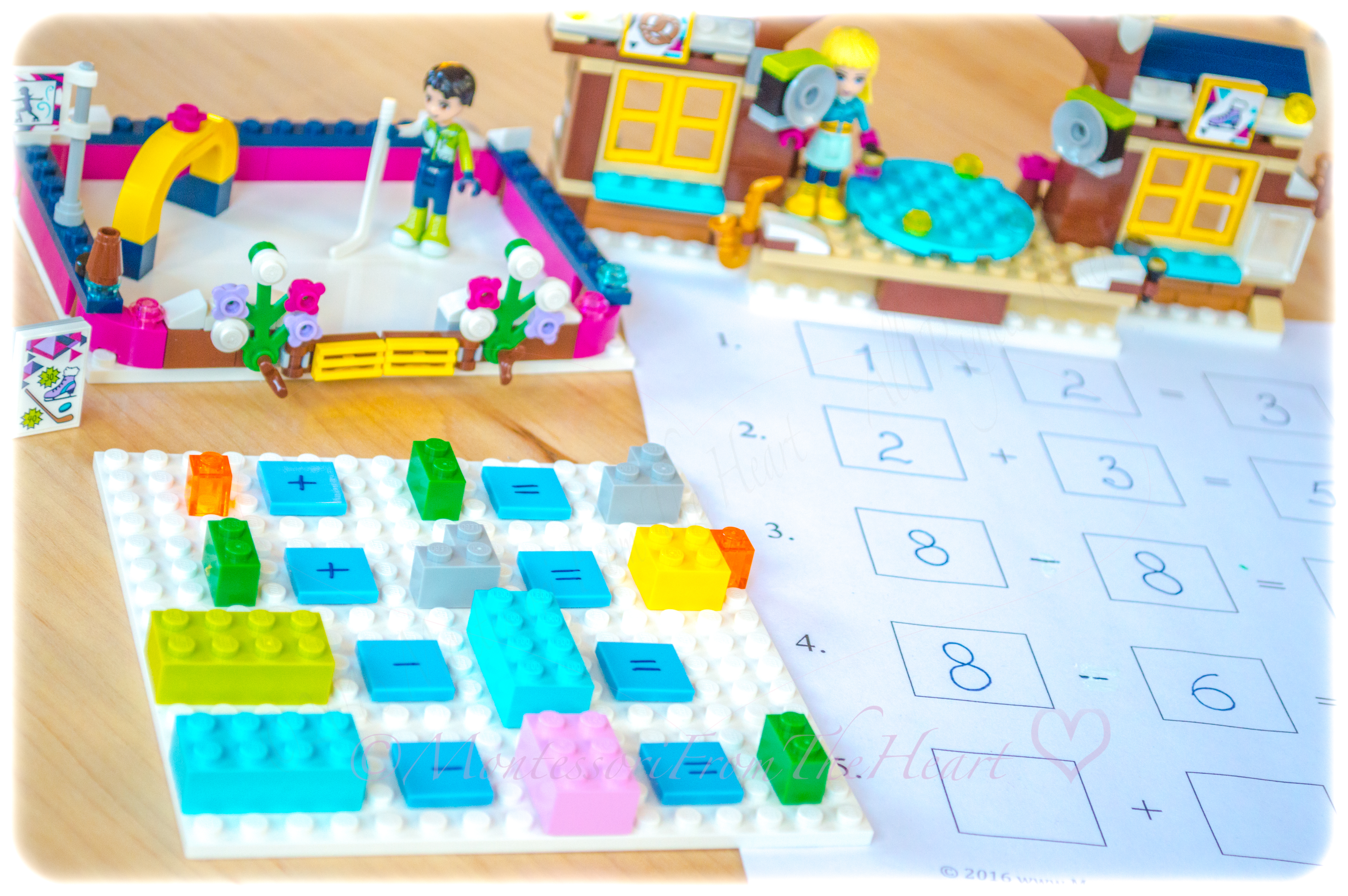After observing Julia doing multiplication exercises, Adrian expressed an interest, so we have been doing some multiplication work this past month. To illustrate the process concretely, we are using these mega building blocks to introduce the simplest concept ✖ 1️⃣~ one times."Times" ✖ means how many times you repeat ~ add~ that number. E.g. 1 x 3 =1+1+1I have written simple multiplication equations on pieces of paper as well as their products (answers). Single mega building blocks illustrated 🙌🏻hands-on how to convert 🤔confusing multiplication into simple➕addition work, which is very familiar to Adrian.In English to "commute" means to travel or to change location. Similarly, in math, the commutative property of multiplication means that two numbers can be multiplied in either order, allowing us to change the places of factors in a product. This means that 1 x 3 = 3 x 1 ~ The product remains the same, despite the factors changing places.Our LEGO DUPLO friends help us multiply. (Buy similar figures here.)Introducing terminology: Factor x Factor = ProductAdrian placed the correct "product" sticker (answer) on its LEGO friend's head.For more on math & LEGO, see here 🔢Math Simple ➕Addition & ➖Subtractions with LEGO.For fractions work, see here Learning Fractions with LEGO.